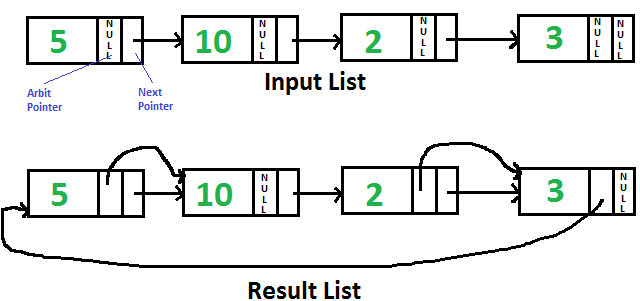# Python Program For Pointing To Next Higher Value Node In A Linked List With An Arbitrary Pointer

• Last Updated : 18 May, 2022

Given singly linked list with every node having an additional “arbitrary” pointer that currently points to NULL. Need to make the “arbitrary” pointer point to the next higher value node.We strongly recommend to minimize your browser and try this yourself first

A Simple Solution is to traverse all nodes one by one, for every node, find the node which has the next greater value of the current node and change the next pointer. Time Complexity of this solution is O(n2).

An Efficient Solution works in O(nLogn) time. The idea is to use Merge Sort for linked list
1) Traverse input list and copy next pointer to arbit pointer for every node.
2) Do Merge Sort for the linked list formed by arbit pointers.

Below is the implementation of the above idea. All of the merger sort functions are taken from here. The taken functions are modified here so that they work on arbit pointers instead of next pointers.

## Python3

 `# Python3 program to populate arbit pointers  ` `# to next higher value using merge sort ` `head ``=` `None` ` `  `# Link l node  ` `class` `Node: ` `     `  `    ``def` `__init__(``self``, data):         ` `        ``self``.data ``=` `data ` `        ``self``.``next` `=` `None` `        ``self``.arbit ``=` `None` ` `  `# Utility function to print result  ` `# linked l ` `def` `printList(node, anode): ` ` `  `    ``print``(``"Traversal using Next Pointer"``) ` `     `  `    ``while` `(node !``=` `None``): ` `        ``print``(node.data, end ``=` `", "``) ` `        ``node ``=` `node.``next` `     `  `    ``print``(``"Traversal using Arbit Pointer"``); ` ` `  `    ``while` `(anode !``=` `None``): ` `        ``print``(anode.data, end ``=` `", "``) ` `        ``anode ``=` `anode.arbit ` `     `  `# This function populates arbit pointer in  ` `# every node to the next higher value. And ` `# returns pointer to the node with minimum ` `# value ` `def` `populateArbit(start): ` ` `  `    ``temp ``=` `start ` ` `  `    ``# Copy next pointers to arbit pointers ` `    ``while` `(temp !``=` `None``): ` `        ``temp.arbit ``=` `temp.``next` `        ``temp ``=` `temp.``next` ` `  `    ``# Do merge sort for arbitrary pointers and ` `    ``# return head of arbitrary pointer linked l ` `    ``return` `MergeSort(start) ` ` `  `# Sorts the linked l formed by arbit pointers  ` `# (does not change next pointer or data) ` `def` `MergeSort(start): ` `     `  `    ``# Base case -- length 0 or 1  ` `    ``if` `(start ``=``=` `None` `or` `start.arbit ``=``=` `None``): ` `        ``return` `start ` ` `  `    ``# Split head into 'middle' and ` `    ``# 'nextofmiddle' sublists  ` `    ``middle ``=` `getMiddle(start) ` `    ``nextofmiddle ``=` `middle.arbit ` `    ``middle.arbit ``=` `None` ` `  `    ``# Recursively sort the sublists  ` `    ``left ``=` `MergeSort(start) ` `    ``right ``=` `MergeSort(nextofmiddle) ` ` `  `    ``# answer = merge the two sorted lists together  ` `    ``sortedlist ``=` `SortedMerge(left, right) ` ` `  `    ``return` `sortedlist ` ` `  `# Utility function to get the  ` `# middle of the linked l ` `def` `getMiddle(source): ` ` `  `    ``# Base case ` `    ``if` `(source ``=``=` `None``): ` `        ``return` `source ` `         `  `    ``fastptr ``=` `source.arbit ` `    ``slowptr ``=` `source ` ` `  `    ``# Move fastptr by two and slow ptr by one  ` `    ``# Finally slowptr will point to middle node ` `    ``while` `(fastptr !``=` `None``): ` `        ``fastptr ``=` `fastptr.arbit ` `         `  `        ``if` `(fastptr !``=` `None``): ` `            ``slowptr ``=` `slowptr.arbit ` `            ``fastptr ``=` `fastptr.arbit ` `         `  `    ``return` `slowptr ` ` `  `def` `SortedMerge(a, b): ` ` `  `    ``result ``=` `None` ` `  `    ``# Base cases  ` `    ``if` `(a ``=``=` `None``): ` `        ``return` `b ` `    ``elif` `(b ``=``=` `None``): ` `        ``return` `a ` ` `  `    ``# Pick either a or b, and recur  ` `    ``if` `(a.data <``=` `b.data): ` `        ``result ``=` `a ` `        ``result.arbit ``=` `SortedMerge(a.arbit, b) ` `    ``else``: ` `        ``result ``=` `b ` `        ``result.arbit ``=` `SortedMerge(a, b.arbit) ` `     `  `    ``return` `result ` ` `  `# Driver code ` `if` `__name__``=``=``'__main__'``: ` `     `  `    ``# Let us create the l shown above  ` `    ``head ``=` `Node(``5``) ` `    ``head.``next` `=` `Node(``10``) ` `    ``head.``next``.``next` `=` `Node(``2``) ` `    ``head.``next``.``next``.``next` `=` `Node(``3``) ` ` `  `    ``# Sort the above created Linked List  ` `    ``ahead ``=` `populateArbit(head) ` ` `  `    ``print``(``"Result Linked List is:"``) ` `    ``printList(head, ahead) ` ` `  `# This code is contributed by rutvik_56  `

Output:

```Result Linked List is:
Traversal using Next Pointer
5, 10, 2, 3,
Traversal using Arbit Pointer
2, 3, 5, 10,```

Please refer complete article on Point to next higher value node in a linked list with an arbitrary pointer for more details!

My Personal Notes arrow_drop_up
Recommended Articles
Page :